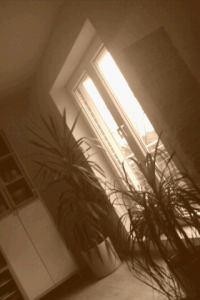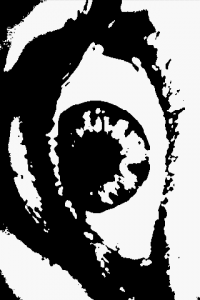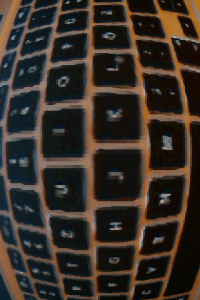# Camera image->NDK->OpenGL texture

Since we are currently working on some augmented reality stuff for Android I need to show the camera image using OpenGL ES. It works great with pure Java if one uses only the grayscale image. However, I needed the color image. The G1’s camera delivers the image in a YUV format while OpenGL only understand RGB images. Unfortunately it is out of question to convert the YUV image to RGB in pure Java for images with 480×320 pixels. Thus, I used the NDK to implement the conversion. The code below does the job. It is based on code provided by Tom Gibara.

```void toRGB565(unsigned short *yuvs, int widthIn, int heightIn, unsigned int *rgbs, int widthOut, int heightOut) {
int half_widthIn = widthIn >> 1;

//the end of the luminance data
int lumEnd = (widthIn * heightIn) >> 1;
//points to the next luminance value pair
int lumPtr = 0;
//points to the next chromiance value pair
int chrPtr = lumEnd;
//the end of the current luminance scanline
int lineEnd = half_widthIn;

int x,y;
for (y=0;y> 1;
for (x=0;x> 8) & 0xff;
Y1 = Y1 & 0xff;
int Cr = yuvs[chrPtr++];
int Cb = ((Cr >> 8) & 0xff) - 128;
Cr = (Cr & 0xff) - 128;

int R, G, B;
//generate first RGB components
B = Y1 + ((454 * Cb) >> 8);
if (B < 0) B = 0; if (B > 255) B = 255;
G = Y1 - ((88 * Cb + 183 * Cr) >> 8);
if (G < 0) G = 0; if (G > 255) G = 255;
R = Y1 + ((359 * Cr) >> 8);
if (R < 0) R = 0; if (R > 255) R = 255;
int val = ((R & 0xf8) << 8) | ((G & 0xfc) << 3) | (B >> 3);

//generate second RGB components
B = Y1 + ((454 * Cb) >> 8);
if (B < 0) B = 0; if (B > 255) B = 255;
G = Y1 - ((88 * Cb + 183 * Cr) >> 8);
if (G < 0) G = 0; if (G > 255) G = 255;
R = Y1 + ((359 * Cr) >> 8);
if (R < 0) R = 0; if (R > 255) R = 255;
rgbs[yPosOut+x] = val | ((((R & 0xf8) << 8) | ((G & 0xfc) << 3) | (B >> 3)) << 16);
}
//skip back to the start of the chromiance values when necessary
chrPtr = lumEnd + ((lumPtr  >> 1) / half_widthIn) * half_widthIn;
lineEnd += half_widthIn;
}
}
```

The code is not that optimized at the moment but can process a 480×320 image in ~25ms on my G1 (which is somewhat slow according to my student’s comments). In order to call this function from Java I needed a wrapper with a JNI signature:

```/**
* Converts the input image from YUV to a RGB 5_6_5 image.
* The size of the output buffer must be at least the size of the input image.
*/
JNIEXPORT void JNICALL Java_de_offis_magic_core_NativeWrapper_image2TextureColor
(JNIEnv *env, jclass clazz,
jbyteArray imageIn, jint widthIn, jint heightIn,
jobject imageOut, jint widthOut, jint heightOut,
jint filter) {

jbyte *cImageIn = (*env)->GetByteArrayElements(env, imageIn, NULL);